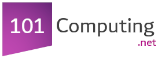=
Assignment operator
AND, OR,
NOT

Boolean operators
/
Divide by (Arithmetic operator)
*
Multiply by (Arithmetic operator)
+
-
Take away (Arithmetic operator)
<
is lower than (Comparison operator)
>
is greater than (Comparison operator)
<=
is lower or equal to (Comparison operator)
>=
is greater or equal to (Comparison operator)
==
is equal to (Comparison operator)
!=
is not equal to (Comparison operator)